In an experiment on photoelectric effect the frequency f of the incident light is plotted against the stopping potential ${\mathrm{V}}_{0}$. The work function of the photoelectric surface is given by (e is electronic charge)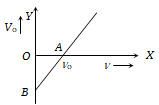(a) OB$×$e in eV
(b) OB in volt
(c) OA in eV
(d) The slope of the line AB

Concept Questions :-

Einstein photo-electric equation
High Yielding Test Series + Question Bank - NEET 2020

Difficulty Level:

The stopping potential as a function of the frequency of the incident radiation is plotted for two different photoelectric surfaces A and B. The graphs show that work function of A is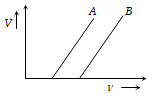(a) Greater than that of B
(b) Smaller than that of B
(c) Equal to that of B
(d) No inference can be drawn about their work functions from the given graphs

Concept Questions :-

Photoelectric effect experiment
High Yielding Test Series + Question Bank - NEET 2020

Difficulty Level:

The graph between intensity of light falling on a metallic plate (I) with the current (i) generated is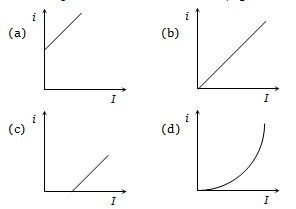Concept Questions :-

Photoelectric effect experiment
High Yielding Test Series + Question Bank - NEET 2020

Difficulty Level:

For a photoelectric cell the graph showing the variation of cut of voltage (${\mathrm{V}}_{0}$) with frequency (v) of incident light is best represented by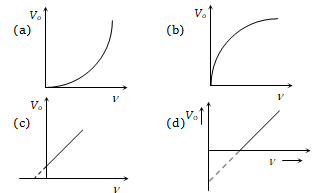Concept Questions :-

Photoelectric effect experiment
High Yielding Test Series + Question Bank - NEET 2020

Difficulty Level:

The correct curve between the stopping potential (V) and intensity of incident light (I) is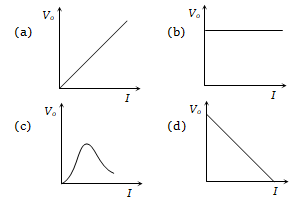Concept Questions :-

Photoelectric effect experiment
High Yielding Test Series + Question Bank - NEET 2020

Difficulty Level:

The value of stopping potential in the following diagram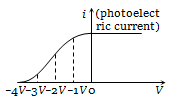(a) – 4V
(b) – 3 V
(c) – 2V
(d) – 1 V

Concept Questions :-

Photoelectric effect experiment
High Yielding Test Series + Question Bank - NEET 2020

Difficulty Level:

In the following diagram if ${\mathrm{V}}_{2}>{\mathrm{V}}_{1}$, then,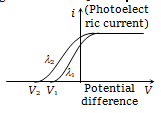(a) ${\mathrm{\lambda }}_{1}=\sqrt{{\mathrm{\lambda }}_{2}}$

(b) ${\mathrm{\lambda }}_{1}<{\mathrm{\lambda }}_{2}$

(c) ${\mathrm{\lambda }}_{1}={\mathrm{\lambda }}_{2}$

(d) ${\mathrm{\lambda }}_{1}>{\mathrm{\lambda }}_{2}$

Concept Questions :-

Photoelectric effect experiment
High Yielding Test Series + Question Bank - NEET 2020

Difficulty Level:

A point source of light is used in an experiment on photoelectric effect. Which of the following curves best represents the variation of photo current (i) with distance (d) of the source from the emitter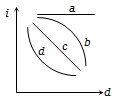(a) a
(b) b
(c) c
(d) d

Concept Questions :-

Photoelectric effect experiment
High Yielding Test Series + Question Bank - NEET 2020

Difficulty Level:

The stopping potential $\left({\mathrm{V}}_{0}\right)$ versus frequency (v) plot of a substance is shown in figure the threshold wave length is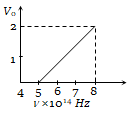(a)

(b) 6000Å

(c) 5000 Å

(d) Can not be estimated from given data

Concept Questions :-

De-broglie wavelength
High Yielding Test Series + Question Bank - NEET 2020

Difficulty Level:

Figure represents a graph of kinetic energy (K) of photoelectrons (in eV) and frequency (v) for a metal used as cathode in photoelectric experiment. The work function of metal is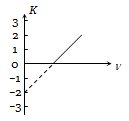(a) 1 eV

(b) 1.5 eV

(c) 2 eV

(d) 3 eV

Concept Questions :-

Einstein photo-electric equation